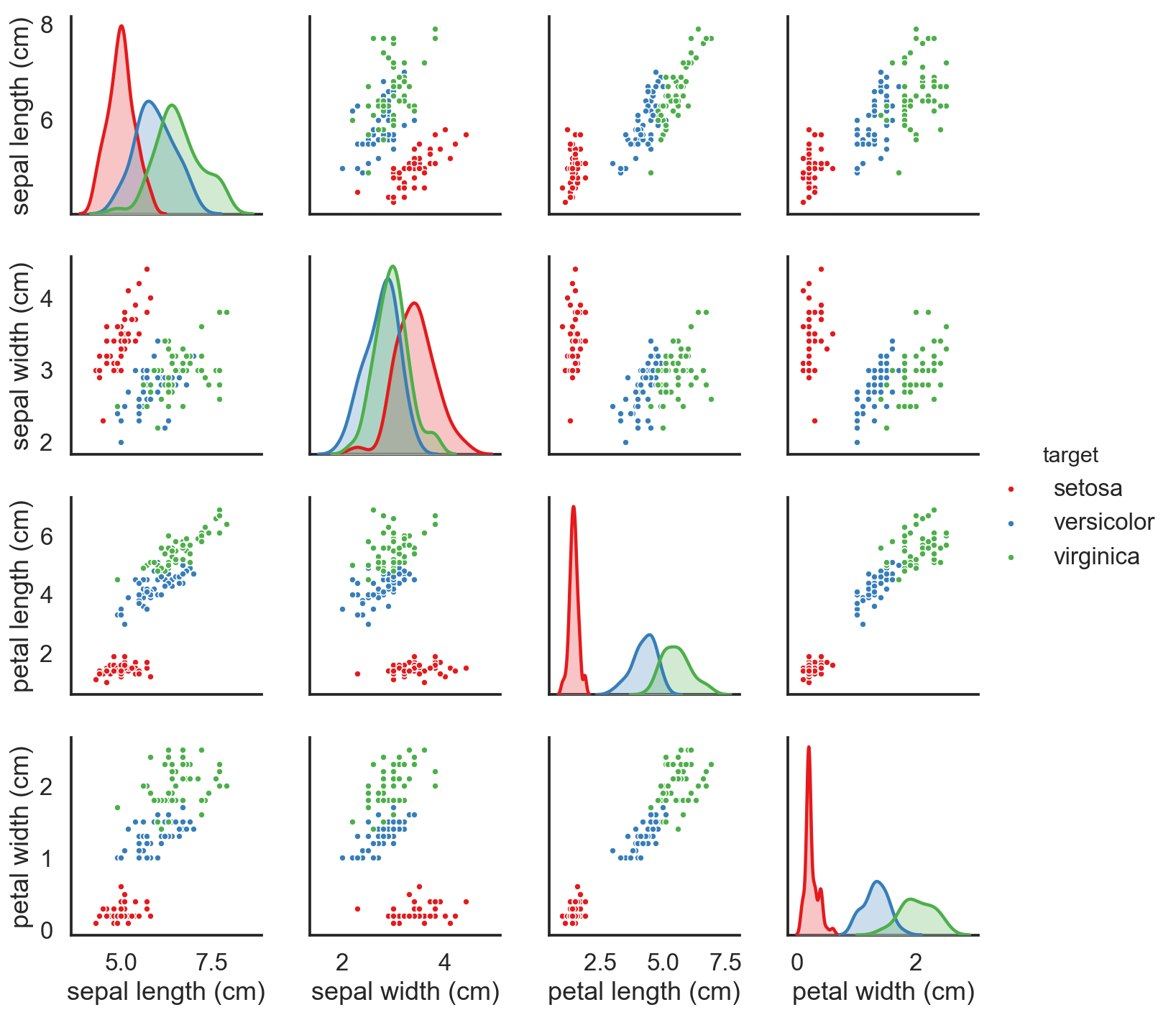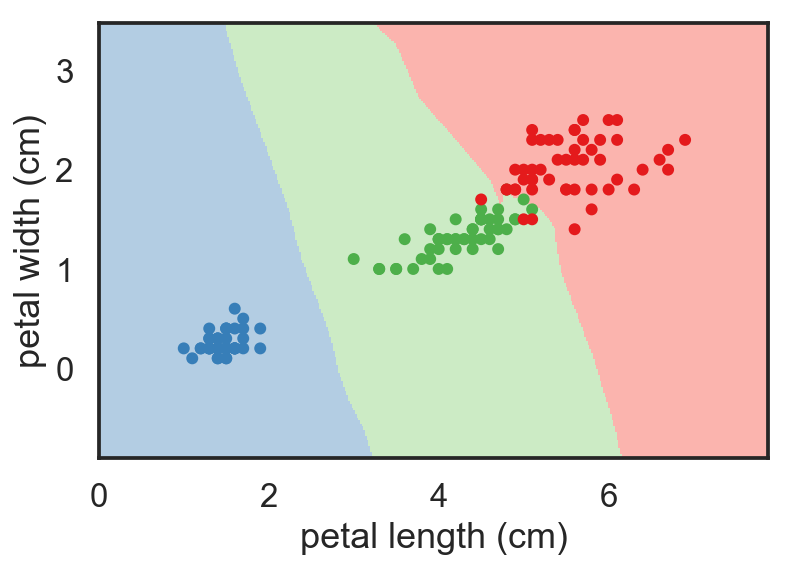# Machine learning with Scikit-learn¶

## Yoav Ram¶

In :
%matplotlib inline
import matplotlib.pyplot as plt
import numpy as np
import pandas as pd
import seaborn as sns
sns.set(
style='white',
context='talk',
palette='Set1'
)Scikit-learn is a Python package for machine learning:

• Simple and efficient tools for data mining and data analysis
• Accessible to everybody, and reusable in various contexts
• Built on NumPy, SciPy, and matplotlib
• Open source, commercially usable - BSD license

We will do one of the many tutorials from the scikit-learn website.

You can install scikit-learn with conda install scikit-learn.

## Supervised learning: predicting an output variable from high-dimensional observations¶

Supervised learning consists in learning the link between two datasets: the observed data X and an external variable y that we are trying to predict, usually called “target” or “labels”. Most often, y is a 1D array of length n_samples.

All supervised estimators in scikit-learn implement a fit(X, y) method to fit the model and a predict(X) method that, given unlabeled observations X, returns the predicted labels y.

## Iris dataset¶

Fisher's Iris dataset is a classification task consisting in identifying 3 different types of irises (Setosa, Versicolour, and Virginica) from their petal and sepal length and width.Let's start by loading the dataset.

In :
import sklearn.datasets

In :
iris = sklearn.datasets.load_iris()
print("Features:", iris.feature_names)
print("Types:", iris.target_names)

Features: ['sepal length (cm)', 'sepal width (cm)', 'petal length (cm)', 'petal width (cm)']
Types: ['setosa' 'versicolor' 'virginica']

In :
df = pd.DataFrame(data=iris.data, columns=iris.feature_names)
df['target'] = iris.target_names[iris.target]
df.head()

Out:
sepal length (cm) sepal width (cm) petal length (cm) petal width (cm) target
0 5.1 3.5 1.4 0.2 setosa
1 4.9 3.0 1.4 0.2 setosa
2 4.7 3.2 1.3 0.2 setosa
3 4.6 3.1 1.5 0.2 setosa
4 5.0 3.6 1.4 0.2 setosa

## K-nearest neighbors classifier (KNN)¶

The simplest possible classifier is the nearest neighbor: given a new observation X_test, find in the training set (i.e. the data used to train the estimator) the observation with the closest feature vector.

Training set and testing set. While experimenting with any learning algorithm, it is important not to test the prediction of an estimator on the data used to fit the estimator as this would not be evaluating the performance of the estimator on new data. This is why datasets are often split into train and test data.

Split the dataset to train and test data using a random permutation - this is easily done with functions from the model_selection module, which has many methods to split datasets. We'll use a very simple one, train_test_split which just splits that data by sampling a fraction of the rows to the training set and the rest to the test set (without replacement).

In :
from sklearn.model_selection import train_test_split

In :
X = iris.data
y = iris.target
X_train, X_test, y_train, y_test = train_test_split(X, y, test_size=0.33, random_state=0)


Import the nearest-neighbor classifier, then create and fit it:

In :
import sklearn.neighbors as nb

In :
knn = nb.KNeighborsClassifier()
knn.fit(X_train, y_train)

Out:
KNeighborsClassifier(algorithm='auto', leaf_size=30, metric='minkowski',
metric_params=None, n_jobs=None, n_neighbors=5, p=2,
weights='uniform')

Predict the labels (Iris species) for the test data and compare with the real labels:

In :
y_hat = knn.predict(X_test)
print(y_hat)
print(y_test)
print('Accuracy:', (y_hat == y_test).mean())

[2 1 0 2 0 2 0 1 1 1 2 1 1 1 1 0 1 1 0 0 2 1 0 0 2 0 0 1 1 0 2 1 0 2 2 1 0
2 1 1 2 0 2 0 0 1 2 2 2 2]
[2 1 0 2 0 2 0 1 1 1 2 1 1 1 1 0 1 1 0 0 2 1 0 0 2 0 0 1 1 0 2 1 0 2 2 1 0
1 1 1 2 0 2 0 0 1 2 2 2 2]
Accuracy: 0.98


# Reduce dimensions¶

To reduce the dimensionality of the problem (4 features - 4D) we can use Seaborn's PairGrid plot to look at the joint distributions of each pair of features.

In :
sns.pairplot(df, hue='target', plot_kws={'s': 20});

/Users/yoavram/miniconda3/envs/DataSciPy/lib/python3.6/site-packages/scipy/stats/stats.py:1713: FutureWarning: Using a non-tuple sequence for multidimensional indexing is deprecated; use arr[tuple(seq)] instead of arr[seq]. In the future this will be interpreted as an array index, arr[np.array(seq)], which will result either in an error or a different result.
return np.add.reduce(sorted[indexer] * weights, axis=axis) / sumvalFrom this figure it seems like using just the petal (last two columns in our features matrix) will do a produce a good separation between blue and others, and a decent one between green and red.

Let's try it.

In :
X_train = X_train[:, 2:]
X_test = X_test[:, 2:]


Fit and predict:

In :
knn = nb.KNeighborsClassifier()
knn.fit(X_train, y_train)
y_hat = knn.predict(X_test)
print(y_hat)
print(y_test)
print('Accuracy:', (y_hat == y_test).mean())

[2 1 0 2 0 2 0 1 1 1 2 1 1 1 1 0 1 1 0 0 2 1 0 0 2 0 0 1 1 0 2 1 0 2 2 1 0
1 1 1 2 0 2 0 0 1 2 2 1 2]
[2 1 0 2 0 2 0 1 1 1 2 1 1 1 1 0 1 1 0 0 2 1 0 0 2 0 0 1 1 0 2 1 0 2 2 1 0
1 1 1 2 0 2 0 0 1 2 2 2 2]
Accuracy: 0.98


We didn't gained any accuracy, but that's expected as the test set size is just 50. But we are in 2D we can plot the classifier fit:

In :
from matplotlib.colors import ListedColormap

h = .02  # step size in the mesh
X = iris.data[:,2:]
y = iris.target

# Create color maps
cmap_light = ListedColormap(sorted(sns.color_palette('Pastel1', 3)))
cmap_bold = ListedColormap(sorted(sns.color_palette('Set1', 3)))

# Plot the decision boundary. For that, we will assign a color to each
# point in the mesh [x_min, m_max]x[y_min, y_max].
x_min, x_max = X[:, 0].min() - 1, X[:, 0].max() + 1
y_min, y_max = X[:, 1].min() - 1, X[:, 1].max() + 1
xx, yy = np.meshgrid(
np.arange(x_min, x_max, h),
np.arange(y_min, y_max, h)
)
Z = knn.predict(np.c_[xx.ravel(), yy.ravel()])

# Put the result into a color plot
Z = Z.reshape(xx.shape)
fig, ax = plt.subplots()
ax.pcolormesh(xx, yy, Z, cmap=cmap_light)

# Plot also the training points
ax.scatter(X[:, 0], X[:, 1], c=y, cmap=cmap_bold, s=20)
ax.set(
xlim=(xx.min(), xx.max()),
ylim=(yy.min(), yy.max()),
xlabel=iris.feature_names,
ylabel=iris.feature_names
);# Recognizing handwritten digits¶

Learning to recognize handwritten digits with a K-nearest neighbors classifier, inspired by IPython Interactive Computing and Visualization Cookbook.

Start by looking at the data. We'll use IPython's widgets to create a slider so we can move between the > 1500 digits images that are in scikit-learn's datasets package.

In :
X, y = sklearn.datasets.load_digits(return_X_y=True)
X.shape, y.shape

Out:
((1797, 64), (1797,))
In :
from ipywidgets import interact
@interact(idx=(0, X.shape - 1))
def show_digit(idx):
fig, ax = plt.subplots(figsize=(1, 1))
ax.matshow(X[idx].reshape(8, 8), cmap='gray_r')
ax.set(xticks=[], yticks=[])
sns.despine(left=True, bottom=True)In :
X_train, X_test, y_train, y_test = train_test_split(X, y, test_size=0.33, random_state=0)

In :
knn = nb.KNeighborsClassifier()
knn.fit(X_train, y_train)
knn.score(X_test, y_test)

Out:
0.9764309764309764

Can we do any better with a different classifier? Let's try the logistic regression classifer. In this model, we try to estimate the probability $p_k$ that a data sample (image) belongs to class $k$ (digit $k$).

In :
from sklearn import linear_model

In :
logistic = linear_model.LogisticRegression()
logistic.fit(X_train, y_train)
logistic.score(X_test, y_test)

Out:
0.95286195286195285

How about a neural network?

In :
from sklearn import neural_network

In :
nn = neural_network.MLPClassifier(hidden_layer_sizes=(1000, 500))
nn.fit(X_train, y_train)
nn.score(X_test, y_test)

Out:
0.9747474747474747

As you can see, the models all have the same API, which allows us to use them like this:

In :
from sklearn import ensemble
from sklearn import svm

models = [
nb.KNeighborsClassifier(),
linear_model.LogisticRegression(),
neural_network.MLPClassifier(),
neural_network.MLPClassifier(hidden_layer_sizes=(1000, 500)),
ensemble.RandomForestClassifier(n_estimators=10),
ensemble.RandomForestClassifier(n_estimators=100),
ensemble.RandomForestClassifier(n_estimators=1000),
svm.SVC()
]

In :
for model in models:
model.fit(X_train, y_train)
print(model)
print(model.score(X_test, y_test))
print()

KNeighborsClassifier(algorithm='auto', leaf_size=30, metric='minkowski',
metric_params=None, n_jobs=1, n_neighbors=5, p=2,
weights='uniform')
0.976430976431

LogisticRegression(C=1.0, class_weight=None, dual=False, fit_intercept=True,
intercept_scaling=1, max_iter=100, multi_class='ovr', n_jobs=1,
penalty='l2', random_state=None, solver='liblinear', tol=0.0001,
verbose=0, warm_start=False)
0.952861952862

MLPClassifier(activation='relu', alpha=0.0001, batch_size='auto', beta_1=0.9,
beta_2=0.999, early_stopping=False, epsilon=1e-08,
hidden_layer_sizes=(100,), learning_rate='constant',
learning_rate_init=0.001, max_iter=200, momentum=0.9,
nesterovs_momentum=True, power_t=0.5, random_state=None,
shuffle=True, solver='adam', tol=0.0001, validation_fraction=0.1,
verbose=False, warm_start=False)
0.952861952862

MLPClassifier(activation='relu', alpha=0.0001, batch_size='auto', beta_1=0.9,
beta_2=0.999, early_stopping=False, epsilon=1e-08,
hidden_layer_sizes=(1000, 500), learning_rate='constant',
learning_rate_init=0.001, max_iter=200, momentum=0.9,
nesterovs_momentum=True, power_t=0.5, random_state=None,
shuffle=True, solver='adam', tol=0.0001, validation_fraction=0.1,
verbose=False, warm_start=False)
0.969696969697

RandomForestClassifier(bootstrap=True, class_weight=None, criterion='gini',
max_depth=None, max_features='auto', max_leaf_nodes=None,
min_impurity_decrease=0.0, min_impurity_split=None,
min_samples_leaf=1, min_samples_split=2,
min_weight_fraction_leaf=0.0, n_estimators=10, n_jobs=1,
oob_score=False, random_state=None, verbose=0,
warm_start=False)
0.93771043771

RandomForestClassifier(bootstrap=True, class_weight=None, criterion='gini',
max_depth=None, max_features='auto', max_leaf_nodes=None,
min_impurity_decrease=0.0, min_impurity_split=None,
min_samples_leaf=1, min_samples_split=2,
min_weight_fraction_leaf=0.0, n_estimators=100, n_jobs=1,
oob_score=False, random_state=None, verbose=0,
warm_start=False)
0.97138047138

RandomForestClassifier(bootstrap=True, class_weight=None, criterion='gini',
max_depth=None, max_features='auto', max_leaf_nodes=None,
min_impurity_decrease=0.0, min_impurity_split=None,
min_samples_leaf=1, min_samples_split=2,
min_weight_fraction_leaf=0.0, n_estimators=1000, n_jobs=1,
oob_score=False, random_state=None, verbose=0,
warm_start=False)
0.976430976431

SVC(C=1.0, cache_size=200, class_weight=None, coef0=0.0,
decision_function_shape='ovr', degree=3, gamma='auto', kernel='rbf',
max_iter=-1, probability=False, random_state=None, shrinking=True,
tol=0.001, verbose=False)
0.393939393939



## Exercise 1¶

The wine dataset contains 13 features and 3 target labels. Apply one of the classifiers in scikit-learn to this dataset. Fit the classifier and score it.

In :
dataset = sklearn.datasets.load_wine()
print(dataset['DESCR'])

Wine Data Database
====================

Notes
-----
Data Set Characteristics:
:Number of Instances: 178 (50 in each of three classes)
:Number of Attributes: 13 numeric, predictive attributes and the class
:Attribute Information:
- 1) Alcohol
- 2) Malic acid
- 3) Ash
- 4) Alcalinity of ash
- 5) Magnesium
- 6) Total phenols
- 7) Flavanoids
- 8) Nonflavanoid phenols
- 9) Proanthocyanins
- 10)Color intensity
- 11)Hue
- 12)OD280/OD315 of diluted wines
- 13)Proline
- class:
- class_0
- class_1
- class_2

:Summary Statistics:

============================= ==== ===== ======= =====
Min   Max   Mean     SD
============================= ==== ===== ======= =====
Alcohol:                      11.0  14.8    13.0   0.8
Malic Acid:                   0.74  5.80    2.34  1.12
Ash:                          1.36  3.23    2.36  0.27
Alcalinity of Ash:            10.6  30.0    19.5   3.3
Magnesium:                    70.0 162.0    99.7  14.3
Total Phenols:                0.98  3.88    2.29  0.63
Flavanoids:                   0.34  5.08    2.03  1.00
Nonflavanoid Phenols:         0.13  0.66    0.36  0.12
Proanthocyanins:              0.41  3.58    1.59  0.57
Colour Intensity:              1.3  13.0     5.1   2.3
Hue:                          0.48  1.71    0.96  0.23
OD280/OD315 of diluted wines: 1.27  4.00    2.61  0.71
Proline:                       278  1680     746   315
============================= ==== ===== ======= =====

:Missing Attribute Values: None
:Class Distribution: class_0 (59), class_1 (71), class_2 (48)
:Creator: R.A. Fisher
:Donor: Michael Marshall (MARSHALL%PLU@io.arc.nasa.gov)
:Date: July, 1988

This is a copy of UCI ML Wine recognition datasets.
https://archive.ics.uci.edu/ml/machine-learning-databases/wine/wine.data

The data is the results of a chemical analysis of wines grown in the same
region in Italy by three different cultivators. There are thirteen different
measurements taken for different constituents found in the three types of
wine.

Original Owners:

Forina, M. et al, PARVUS -
An Extendible Package for Data Exploration, Classification and Correlation.
Institute of Pharmaceutical and Food Analysis and Technologies,
Via Brigata Salerno, 16147 Genoa, Italy.

Citation:

Lichman, M. (2013). UCI Machine Learning Repository
[http://archive.ics.uci.edu/ml]. Irvine, CA: University of California,
School of Information and Computer Science.

References
----------
(1)
S. Aeberhard, D. Coomans and O. de Vel,
Comparison of Classifiers in High Dimensional Settings,
Tech. Rep. no. 92-02, (1992), Dept. of Computer Science and Dept. of
Mathematics and Statistics, James Cook University of North Queensland.
(Also submitted to Technometrics).

The data was used with many others for comparing various
classifiers. The classes are separable, though only RDA
has achieved 100% correct classification.
(RDA : 100%, QDA 99.4%, LDA 98.9%, 1NN 96.1% (z-transformed data))
(All results using the leave-one-out technique)

(2)
S. Aeberhard, D. Coomans and O. de Vel,
"THE CLASSIFICATION PERFORMANCE OF RDA"
Tech. Rep. no. 92-01, (1992), Dept. of Computer Science and Dept. of
Mathematics and Statistics, James Cook University of North Queensland.
(Also submitted to Journal of Chemometrics).


In [ ]:
X = dataset.data
y = dataset.target

In :


Test accuracy: 0.9778


# Regression¶

We'll work with the diabetes dataset:

Ten baseline variables, age, sex, body mass index, average blood pressure, and six blood serum measurements were obtained for each of n = 442 diabetes patients, as well as the response of interest, a quantitative measure of disease progression one year after baseline.

In :
diabetes = sklearn.datasets.load_diabetes()
X = diabetes.data
y = diabetes.target


Let's look at the features (X):

In :
df = pd.DataFrame(data=X, columns=diabetes.feature_names)
sns.pairplot(df, plot_kws=dict(alpha=0.25));